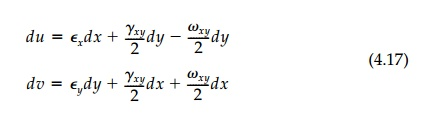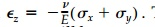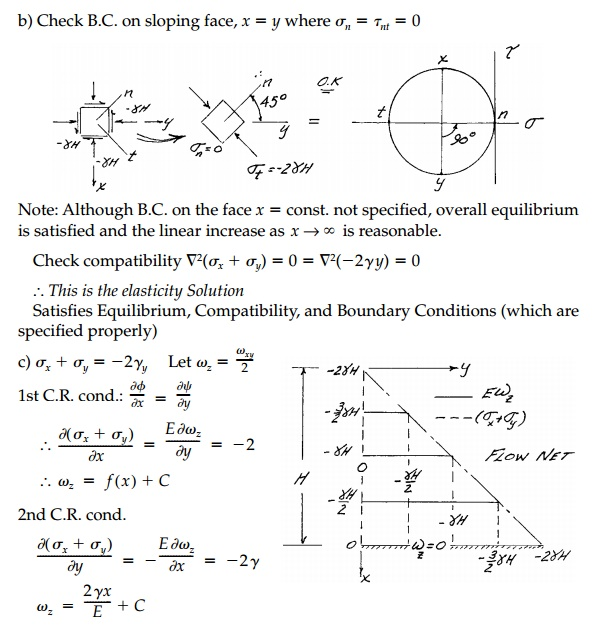Home | | Mechanics of Solids | The Conjugate Relationship Between Mean Stress and Rotation

# The Conjugate Relationship Between Mean Stress and Rotation

The first invariant of the stress or strain tensor, the sum of the normal compo-nents, is harmonic for a simply-connected domain and constant body forces.

The Conjugate Relationship Between Mean Stress and Rotation

The first invariant of the stress or strain tensor, the sum of the normal compo-nents, is harmonic for a simply-connected domain and constant body forces. This in turn implies the existence of a conjugate function, which must simi-larly be harmonic and also invariant to linear tangent transformation.

From experience with the significance of conjugate pairs in other potential fields, we might expect a similar importance to be attached to the conjugate harmonic function in the elastic continuum. This is not so. Occasionally pass-ing reference is made to a conjugate relationship between elastic rotations and the mean stress or strain, but the explicit statement of this correspon-dence is seldom presented.

This is probably because in the standard formulation for simply-connected, two-dimensional problems, the invariant elastic rotation,xy , not correspond-ing directly to stress components, is neglected. The compatibility requirement is then expressed as:Finally by expressing Equation (2.7) in terms of stresses from Equation (2.4) and combining with the equilibrium Equation (2.45), the basic harmonic equations:result whenever the body forces Bx and By are negligible or constant. The meanThus, neglecting body forces, we have the Cauchy-Riemann conditions directly and the conjugate relationship between the elastic rotation of a differ-ential element and the first invariant of the 2D stress tensor is established.

To determine elastic rotations around the x and y axes associated with plane stress and plane strain remember that:Some general observations follow directly from these equations:

1.  For an incompressible material ( v 0.5) in plane strain, Em is zero and the displacements u and v themselves become conjugate har-monic functions.

2.  From Equation (4.12) we can obtain the compatibility equation in its standard form [Equation (2.7)] and derive a second independent relationship:3.  Both the elliptic equations associated with Equation (4.13) are of similar form:and are Laplacian whenever the body forces are negligible or con-stant. In the event the body forces are constant such as in a gravity field, Equation (4.13) can be reduced to the Cauchy-Riemann con-ditions and direct solution achieved by a suitable transformation. For example, if we define:

then from Equation (4.13)and we can again solve for A and B by standard methods.

4.  Direct integration to determine displacements for plane strain (or plane stress in the plane z = 0) is greatly facilitated in that:where now we can determine the rotation from the stress field with Equation 4.13.

5.  Finally for the general 3D case, both the first invariant of the stress or strain tensor:and the invariant mean rotation of a volume element:will also clearly be Laplacian.

In general the conjugate relationships are more complicated in three-dimensional situations. Often, however, as is the case of plane stress [Equa-tion (4.14)] or where there is a high degree of symmetry, the components of rotation can be determined easily, in which case displacements can be found by direct integration of the relative displacement equations:Thus we see that, without body forces, the elastic rotations times E are the conjugate harmonic function to twice the mean stress and that these two sca-lar invariants go 'hand-in-hand' throughout a structure, one controlling the other in accordance with Laplace's equation. If we can solve for one, we have solved for the other, and in that sense it is correct to neglect the rotations in that they will come out of the solution for the stress (or strain) field of their own accord.* This scalar part of the total tensor field giving the conjugate har-monic invariantsq andxy (orm andin 3D) we shall call the 'isotropic field.' Only the center of Mohr's Circle or, physically, pure volume change is involved.

Unfortunately it is seldom possible to specify a complete set of proper boundary conditions in terms ofq orxy for problems in solid mechanics. For example, at most boundaries the tangential normal stress is unknown, and only if the boundary is clamped will there be zero rotation. Thus it is usu-ally not possible to solve for the isotropic field independent of the deviatoric field.

Nevertheless, flow nets are extremely useful:

1.  To depict the isotropic field so it can be understood once known.

2.  Determine the isotropic field if the boundary conditions can be found by other methods such as photoelasticity which givesm on the boundary directly.

3.  As a conceptual tool for optimum design.

A number of isotropic flow nets for important stress fields will be plotted in later chapters and will be called for in chapter problems. Two examples are shown in Figures 4.2 and 4.3 which will be referred to later when the solutions to these important problems are presented. In both these struc-tures, symmetry requires that certain lines do not rotate, which simplifies the construction.

Interferometry provides the best optical full-field method to see, in the lab-oratory, the isopachics-contour lines of 'equal thickness'-which, by Equation (4.6), are proportional to the mean stress. This is to say that, for plane stress, the normal strain. Thus when a model is      loaded, the change in the thickness, t, will beBy interfering with the wave front of light through or reflected off the unstressed model with the wavefront from the stressed model, the change in thickness produces 'fringes' of integer order N, proportional to w, with precision in multiples of the wavelength of light,. More specificallyUntil the advent of the laser, interferometry was extremely expensive and not very successful even in the hands of the best experimentalist. However, laser light is almost perfectly monochromatic and, most importantly, coherent.Thus, model surfaces need not be flat since the reference (the unloaded) light beam can be captured with a hologram. This makes the experimental deter-mination of the isotropic stress field practical and the methodology continues to improve. A reflection holographic interferogram is shown in Figure 4.3 for comparison to the isopachics derived from the analytic solution.

Example 4.1Study Material, Lecturing Notes, Assignment, Reference, Wiki description explanation, brief detail
Civil : Principles of Solid Mechanics : Strategies for Elastic Analysis and Design : The Conjugate Relationship Between Mean Stress and Rotation |

Related Topics